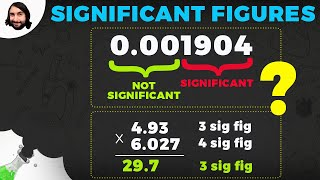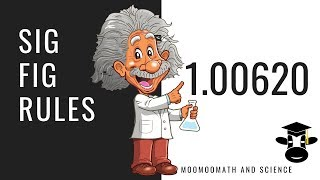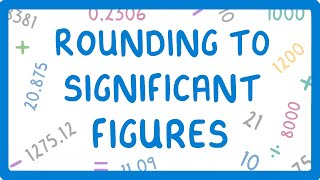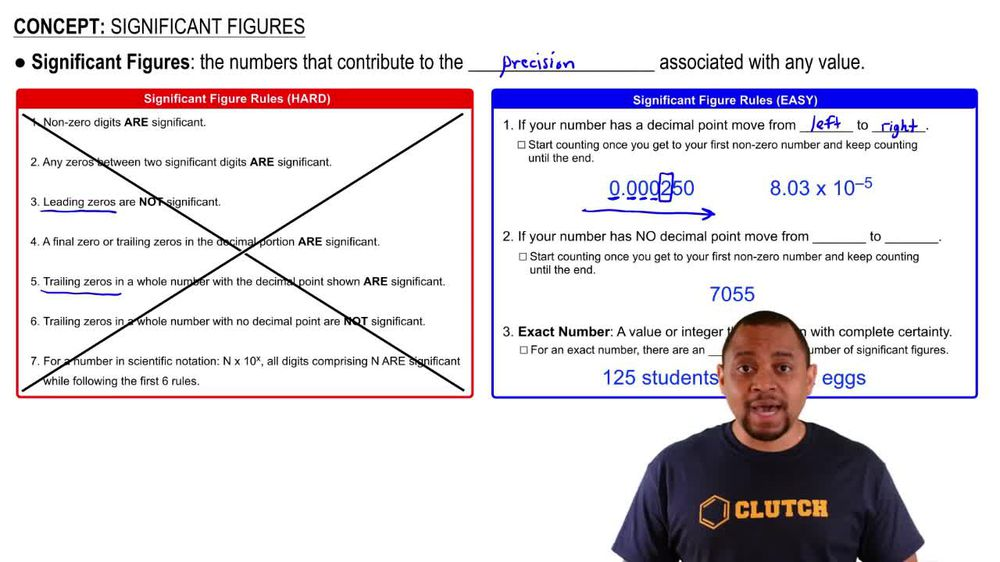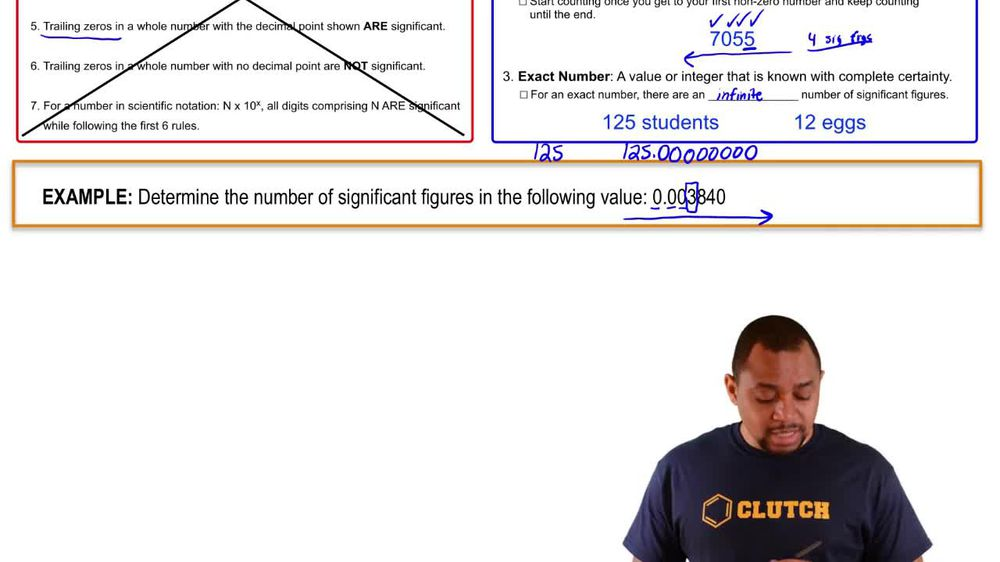Start typing, then use the up and down arrows to select an option from the list.
1. 1. Intro to General Chemistry2. Significant Figures
Problem

# How many significant figures are in each of the following measurements? (d) 510 J

Relevant Solution1m
Play a video:
Hi everyone for this problem, we need to determine the number of significant figures in 180,000 gallons. So in this problem we see that we have some zeros here and a rule to remember when it comes to significant figures and zeros is that zeros that appear, after all, non zero digits are called trailing zeros. And so for this problem, we have four trailing zeros and trailing zeros. In a number that lacks a decimal point are not significant, so we can go ahead and ignore these four zeros because they're not significant. And the question is asking us to determine the number of significant figures. So our answer here is that this number has to significant figures and that is the answer to this question. I hope this was helpful.